Next: Digital Circuits Up: Operational Amplifiers Previous: Current Limiting and Slew

# Problems

1. Consider the circuit below. (You may assume that the op-amps are ideal.)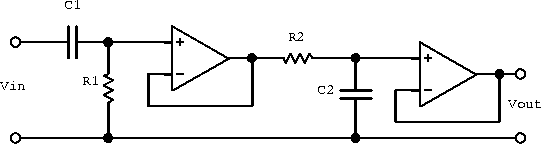1. Write an expression for the transfer function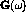. Express your result in terms of the amplitude of the output and the phase relative to the input. Let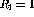k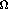,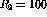,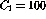F and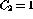F. Do not simplify the algebra.
2. What are the (real) zeroes in the transfer function, if any?
3. What are the (real) poles in the transfer function if any?
4. Sketch the transfer function as a function ofon a log-log plot. Your sketch should show the slope of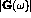in the large and smalllimits, the corner frequencies, and the value ofat the corner frequencies.
5. Describe the dependence of the output on frequency at small and large frequencies in dB/octave.
1. Write the two rules for the analysis of circuits which utilize ``ideal'' op-amps.
2. Write an expression for the potential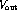for the following circuit.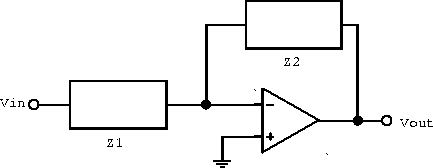3. Letkand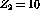k. Sketch a log-log plot showing the functionfor the above circuit assuming that a general purpose op-amp such as the 741 is used.
4. The 741 op-amp has a corner frequencies of 4 Hz, DC open-loop gain of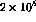and a fall off at high frequency of 6 dB/octave. What is the frequency domain over which the amplifier defined in part (c) will have constant gain? What is the gain of the amplifier in this frequency domain?
5. Suppose now that the impedance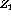is replaced with a capacitor with a capacitance C (k). For frequencies much greater than 4 Hz, the 741 op-amp will attenuate the signal if the product RC is greater than some maximum value. For a frequency of 50 kHz, what is the maximum value for C for which the op-amp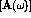will not attenuate the output signal at high frequencies? Hint: If you sketch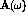and, you will be able to see the constraint on.
2. Set up an operational amplifier circuit to solve the equation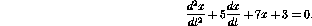Hint: the input is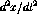.

3. Draw a schematic diagram of a circuit for which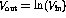. Specify the limitations on the input voltage range, if any.Next: Digital Circuits Up: Operational Amplifiers Previous: Current Limiting and Slew

Doug Gingrich
Tue Jul 13 16:55:15 EDT 1999